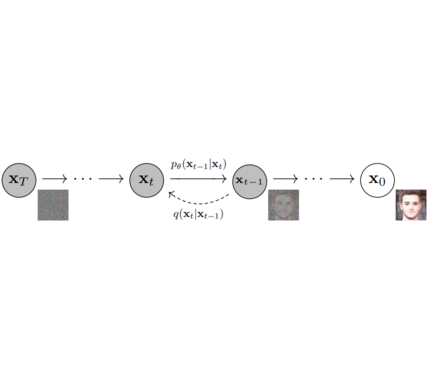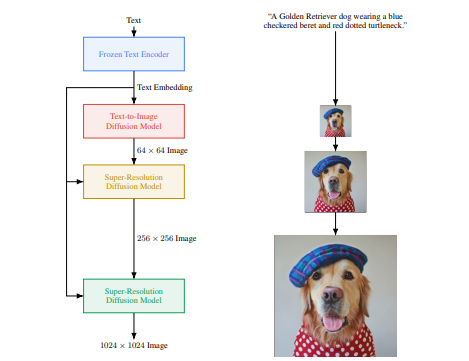🤗 Diffusers provides pretrained diffusion models across multiple modalities, such as vision and audio, and serves as a modular toolbox for inference and training of diffusion models.

More precisely, 🤗 Diffusers offers:

• State-of-the-art diffusion pipelines that can be run in inference with just a couple of lines of code (see src/diffusers/pipelines).
• Various noise schedulers that can be used interchangeably for the prefered speed vs. quality trade-off in inference (see src/diffusers/schedulers).
• Multiple types of models, such as UNet, that can be used as building blocks in an end-to-end diffusion system (see src/diffusers/models).
• Training examples to show how to train the most popular diffusion models (see examples).

## Definitions

Models: Neural network that models p_θ(x_t-1|x_t) (see image below) and is trained end-to-end to denoise a noisy input to an image. Examples: UNet, Conditioned UNet, 3D UNet, Transformer UNetSchedulers: Algorithm class for both inference and training. The class provides functionality to compute previous image according to alpha, beta schedule as well as predict noise for training. Examples: DDPM, DDIM, PNDM, DEIS

Diffusion Pipeline: End-to-end pipeline that includes multiple diffusion models, possible text encoders, … Examples: GLIDE, Latent-Diffusion, Imagen, DALL-E 2## Philosophy

• Readability and clarity is prefered over highly optimized code. A strong importance is put on providing readable, intuitive and elementary code desgin. E.g., the provided schedulers are separated from the provided models and provide well-commented code that can be read alongside the original paper.
• Diffusers is modality independent and focusses on providing pretrained models and tools to build systems that generate continous outputs, e.g. vision and audio.
• Diffusion models and schedulers are provided as consise, elementary building blocks whereas diffusion pipelines are a collection of end-to-end diffusion systems that can be used out-of-the-box, should stay as close as possible to their original implementation and can include components of other library, such as text-encoders. Examples for diffusion pipelines are Glide and Latent Diffusion.

## Quickstart

### Installation

``````pip install diffusers  # should install diffusers 0.0.4
``````

### 1. `diffusers` as a toolbox for schedulers and models.

`diffusers` is more modularized than `transformers`. The idea is that researchers and engineers can use only parts of the library easily for the own use cases. It could become a central place for all kinds of models, schedulers, training utils and processors that one can mix and match for one’s own use case. Both models and schedulers should be load- and saveable from the Hub.

For more examples see schedulers and models

#### Example for DDPM:

```import torch
from diffusers import UNetModel, DDPMScheduler
import PIL
import numpy as np
import tqdm

generator = torch.manual_seed(0)
torch_device = "cuda" if torch.cuda.is_available() else "cpu"

noise_scheduler = DDPMScheduler.from_config("fusing/ddpm-lsun-church", tensor_format="pt")
unet = UNetModel.from_pretrained("fusing/ddpm-lsun-church").to(torch_device)

# 2. Sample gaussian noise
image = torch.randn(
(1, unet.in_channels, unet.resolution, unet.resolution),
generator=generator,
)
image = image.to(torch_device)

# 3. Denoise
num_prediction_steps = len(noise_scheduler)
for t in tqdm.tqdm(reversed(range(num_prediction_steps)), total=num_prediction_steps):
# predict noise residual
residual = unet(image, t)

# predict previous mean of image x_t-1
pred_prev_image = noise_scheduler.step(residual, image, t)

# optionally sample variance
variance = 0
if t > 0:
noise = torch.randn(image.shape, generator=generator).to(image.device)
variance = noise_scheduler.get_variance(t).sqrt() * noise

# set current image to prev_image: x_t -> x_t-1
image = pred_prev_image + variance

# 5. process image to PIL
image_processed = image.cpu().permute(0, 2, 3, 1)
image_processed = (image_processed + 1.0) * 127.5
image_processed = image_processed.numpy().astype(np.uint8)
image_pil = PIL.Image.fromarray(image_processed)

# 6. save image
image_pil.save("test.png")```

#### Example for DDIM:

```import torch
from diffusers import UNetModel, DDIMScheduler
import PIL
import numpy as np
import tqdm

generator = torch.manual_seed(0)
torch_device = "cuda" if torch.cuda.is_available() else "cpu"

noise_scheduler = DDIMScheduler.from_config("fusing/ddpm-celeba-hq", tensor_format="pt")
unet = UNetModel.from_pretrained("fusing/ddpm-celeba-hq").to(torch_device)

# 2. Sample gaussian noise
image = torch.randn(
(1, unet.in_channels, unet.resolution, unet.resolution),
generator=generator,
)
image = image.to(torch_device)

# 3. Denoise
num_inference_steps = 50
eta = 0.0  # <- deterministic sampling

for t in tqdm.tqdm(reversed(range(num_inference_steps)), total=num_inference_steps):
# 1. predict noise residual
orig_t = noise_scheduler.get_orig_t(t, num_inference_steps)
residual = unet(image, orig_t)

# 2. predict previous mean of image x_t-1
pred_prev_image = noise_scheduler.step(residual, image, t, num_inference_steps, eta)

# 3. optionally sample variance
variance = 0
if eta > 0:
noise = torch.randn(image.shape, generator=generator).to(image.device)
variance = noise_scheduler.get_variance(t).sqrt() * eta * noise

# 4. set current image to prev_image: x_t -> x_t-1
image = pred_prev_image + variance

# 5. process image to PIL
image_processed = image.cpu().permute(0, 2, 3, 1)
image_processed = (image_processed + 1.0) * 127.5
image_processed = image_processed.numpy().astype(np.uint8)
image_pil = PIL.Image.fromarray(image_processed)

# 6. save image
image_pil.save("test.png")```

### 2. `diffusers` as a collection of popula Diffusion systems (GLIDE, Dalle, …)

For more examples see pipelines.

#### Example image generation with PNDM

```from diffusers import PNDM, UNetModel, PNDMScheduler
import PIL.Image
import numpy as np
import torch

model_id = "fusing/ddim-celeba-hq"

model = UNetModel.from_pretrained(model_id)
scheduler = PNDMScheduler()

pndm = PNDM(unet=model, noise_scheduler=scheduler)

# run pipeline in inference (sample random noise and denoise)
image = pndm()

# process image to PIL
image_processed = image.cpu().permute(0, 2, 3, 1)
image_processed = (image_processed + 1.0) / 2
image_processed = torch.clamp(image_processed, 0.0, 1.0)
image_processed = image_processed * 255
image_processed = image_processed.numpy().astype(np.uint8)
image_pil = PIL.Image.fromarray(image_processed)

# save image
image_pil.save("test.png")```

#### Text to Image generation with Latent Diffusion

Note: To use latent diffusion install transformers from this branch.

```from diffusers import DiffusionPipeline

ldm = DiffusionPipeline.from_pretrained("fusing/latent-diffusion-text2im-large")

generator = torch.manual_seed(42)

prompt = "A painting of a squirrel eating a burger"
image = ldm([prompt], generator=generator, eta=0.3, guidance_scale=6.0, num_inference_steps=50)

image_processed = image.cpu().permute(0, 2, 3, 1)
image_processed = image_processed  * 255.
image_processed = image_processed.numpy().astype(np.uint8)
image_pil = PIL.Image.fromarray(image_processed)

# save image
image_pil.save("test.png")```

#### Text to speech with BDDM

```import torch
from diffusers import BDDM, DiffusionPipeline

torch_device = "cuda"

bddm = DiffusionPipeline.from_pretrained("fusing/diffwave-vocoder-ljspeech")

# load tacotron2 to get the mel spectograms
tacotron2 = tacotron2.to(torch_device).eval()

text = "Hello world, I missed you so much."

sequences, lengths = utils.prepare_input_sequence([text])

# generate mel spectograms using text
mel_spec, _, _ = tacotron2.infer(sequences, lengths)

# generate the speech by passing mel spectograms to BDDM pipeline
generator = torch.manual_seed(0)
audio = bddm(mel_spec, generator, torch_device)

# save generated audio
from scipy.io.wavfile import write as wavwrite
sampling_rate = 22050
wavwrite("generated_audio.wav", sampling_rate, audio.squeeze().cpu().numpy())```

## TODO

• Create common API for models [ ]
• Add tests for models [ ]
• Adapt schedulers for training [ ]
• Write google colab for training [ ]
• Write docs / Think about how to structure docs [ ]
• Add tests to circle ci [ ]
• Add more vision models [ ]
• Add more speech models [ ]
• Add RL model [ ]

View Github